# RD Sharma Solutions For Class 12 Maths Exercise 5.5 Chapter 5 Algebra of Matrices

RD Sharma Solutions for Class 12 Maths Exercise 5.5 Chapter 5 Algebra of Matrices is provided here. The problems are solved in such a way that they help students understand the topics and improve their problem-solving abilities. Practising lots of examples before solving the main exercise makes them familiar with the formulas and methods.

The RD Sharma Solutions are prepared by experts at BYJU’S with the aim of helping students score well in the board exams. In the CBSE exam pattern each step carries marks, so the answers are prepared with explanations to make it easy for understanding. RD Sharma Solutions for Class 12 Maths Chapter 5 Algebra of Matrices Exercise 5.5 are provided here.

## Download the PDF of RD Sharma Solutions For Class 12 Chapter 5 – Algebra of Matrices Exercise 5.5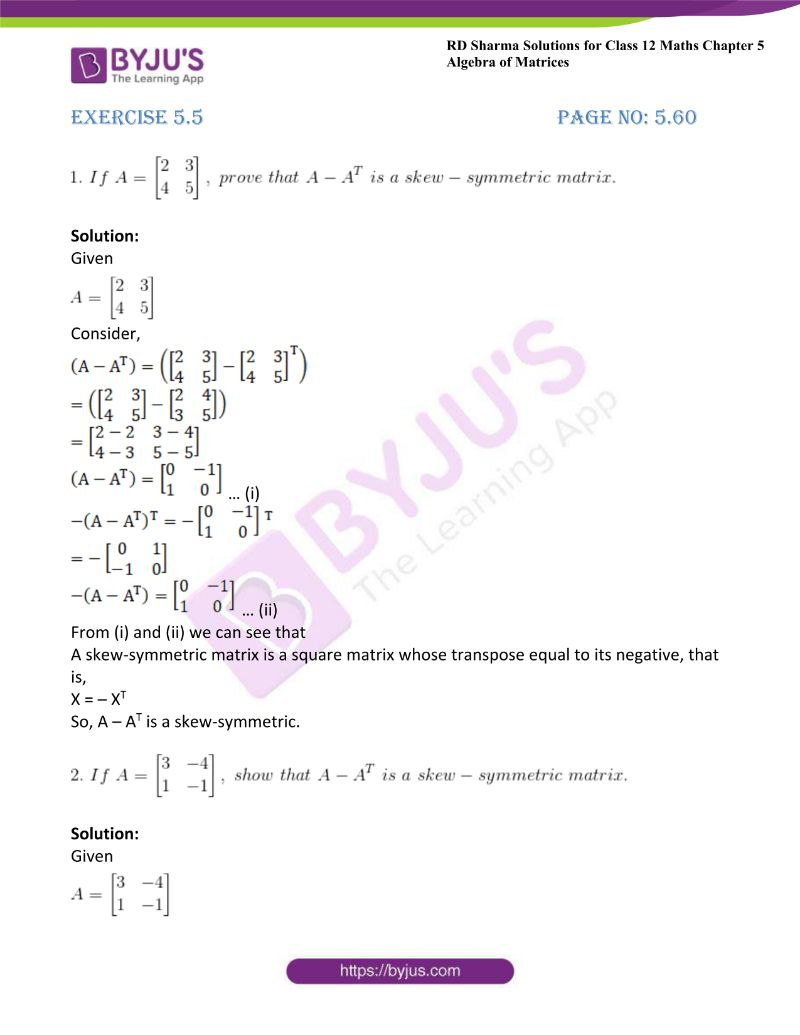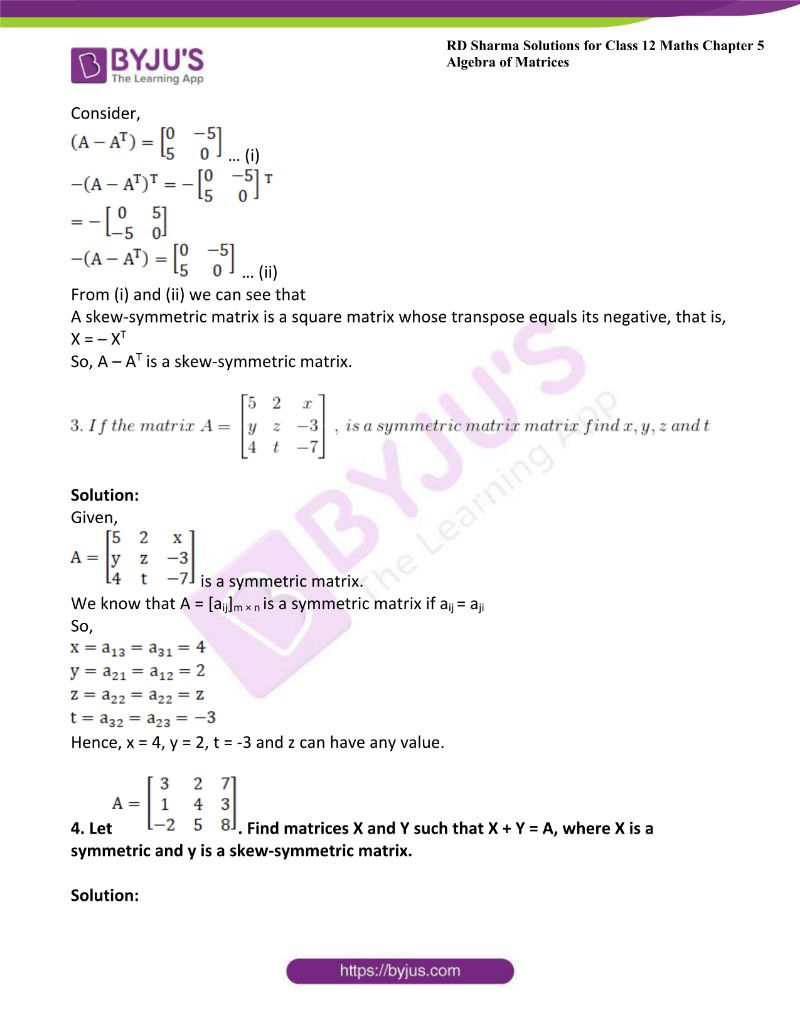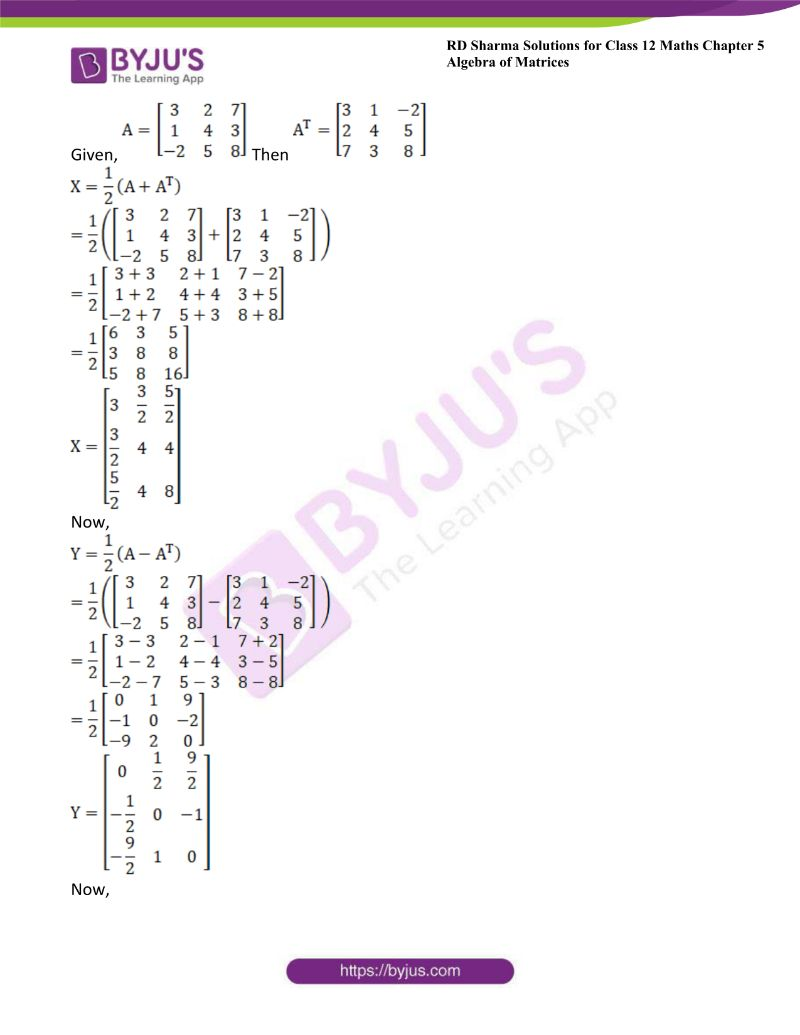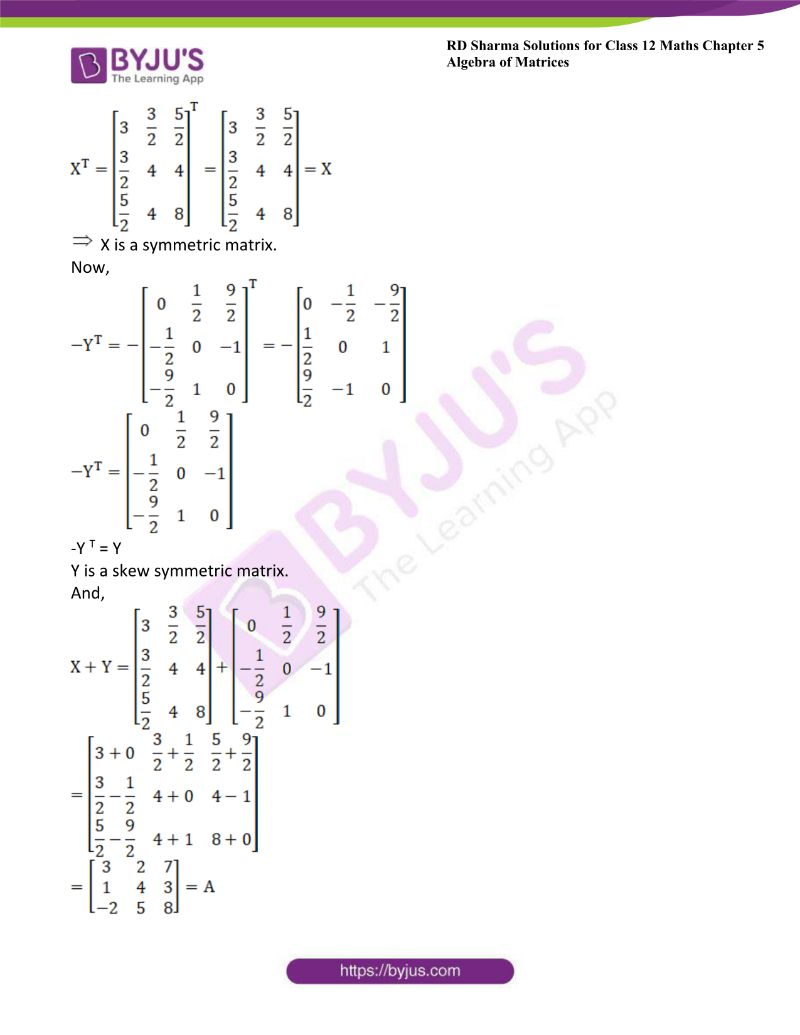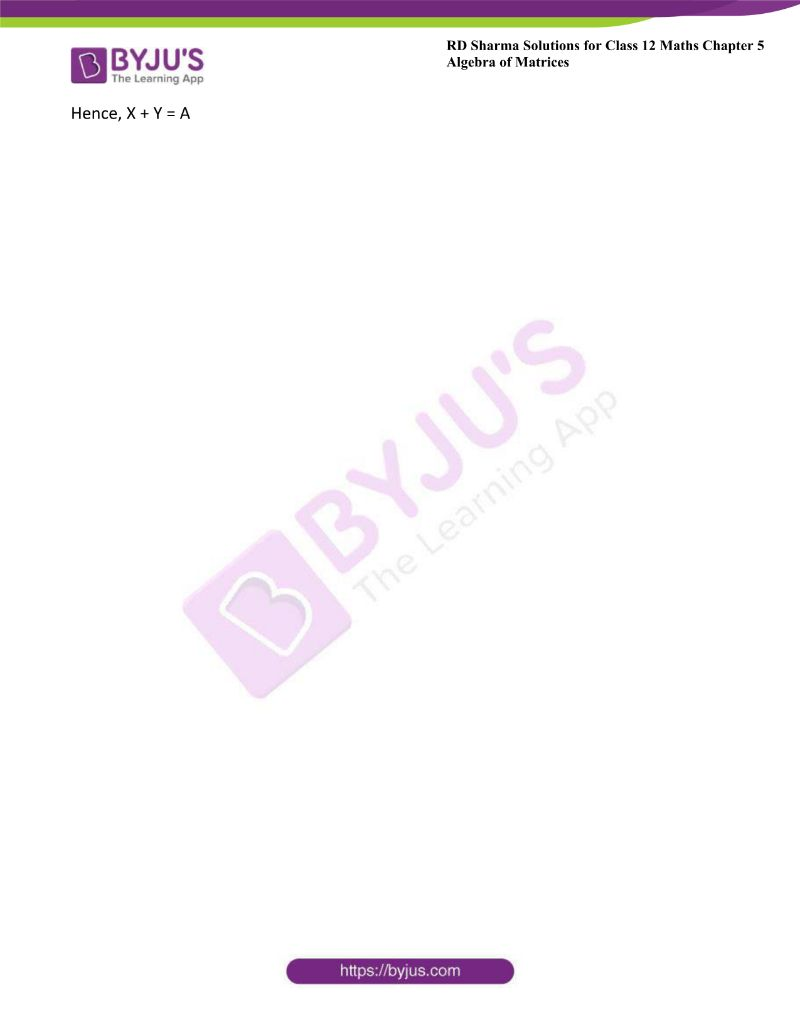### Access other exercises of RD Sharma Solutions For Class 12 Chapter 5 – Algebra of Matrices

Exercise 5.1 Solutions

Exercise 5.2 Solutions

Exercise 5.3 Solutions

Exercise 5.4 Solutions

### Access answers to Maths RD Sharma Solutions For Class 12 Chapter 5 – Algebra of Matrices Exercise 5.5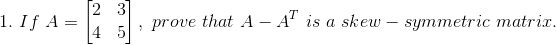Solution:

Given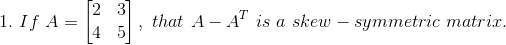Consider,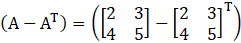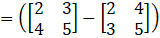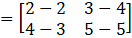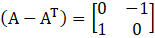… (i)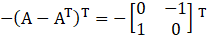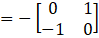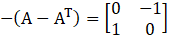… (ii)

From (i) and (ii) we can see that

A skew-symmetric matrix is a square matrix whose transpose equal to its negative, that is,

X = – XT

So, A – AT is a skew-symmetric.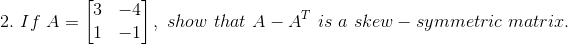Solution:

GivenConsider,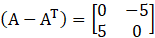… (i)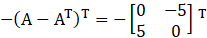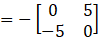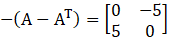… (ii)

From (i) and (ii) we can see that

A skew-symmetric matrix is a square matrix whose transpose equals its negative, that is,

X = – XT

So, A – AT is a skew-symmetric matrix.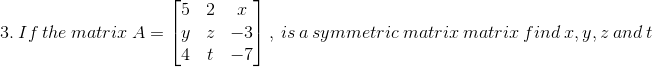Solution:

Given,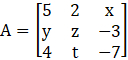is a symmetric matrix.

We know that A = [aij]m × n is a symmetric matrix if aij = aji

So,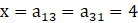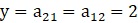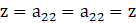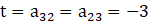Hence, x = 4, y = 2, t = -3 and z can have any value.

4. Let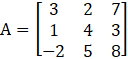. Find matrices X and Y such that X + Y = A, where X is a symmetric and y is a skew-symmetric matrix.

Solution:

Given,Then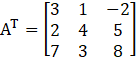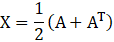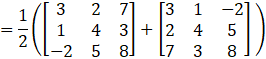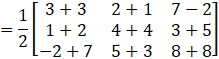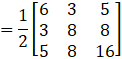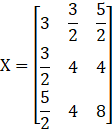Now,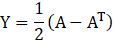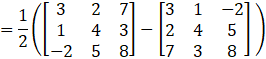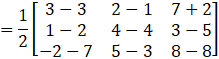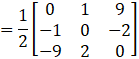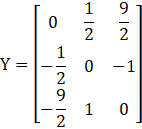Now,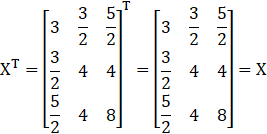X is a symmetric matrix.

Now,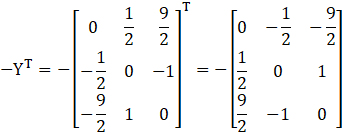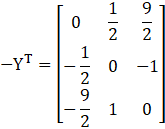-Y T = Y

Y is a skew symmetric matrix.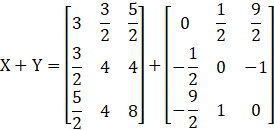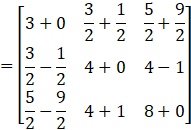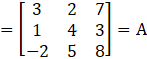Hence, X + Y = A